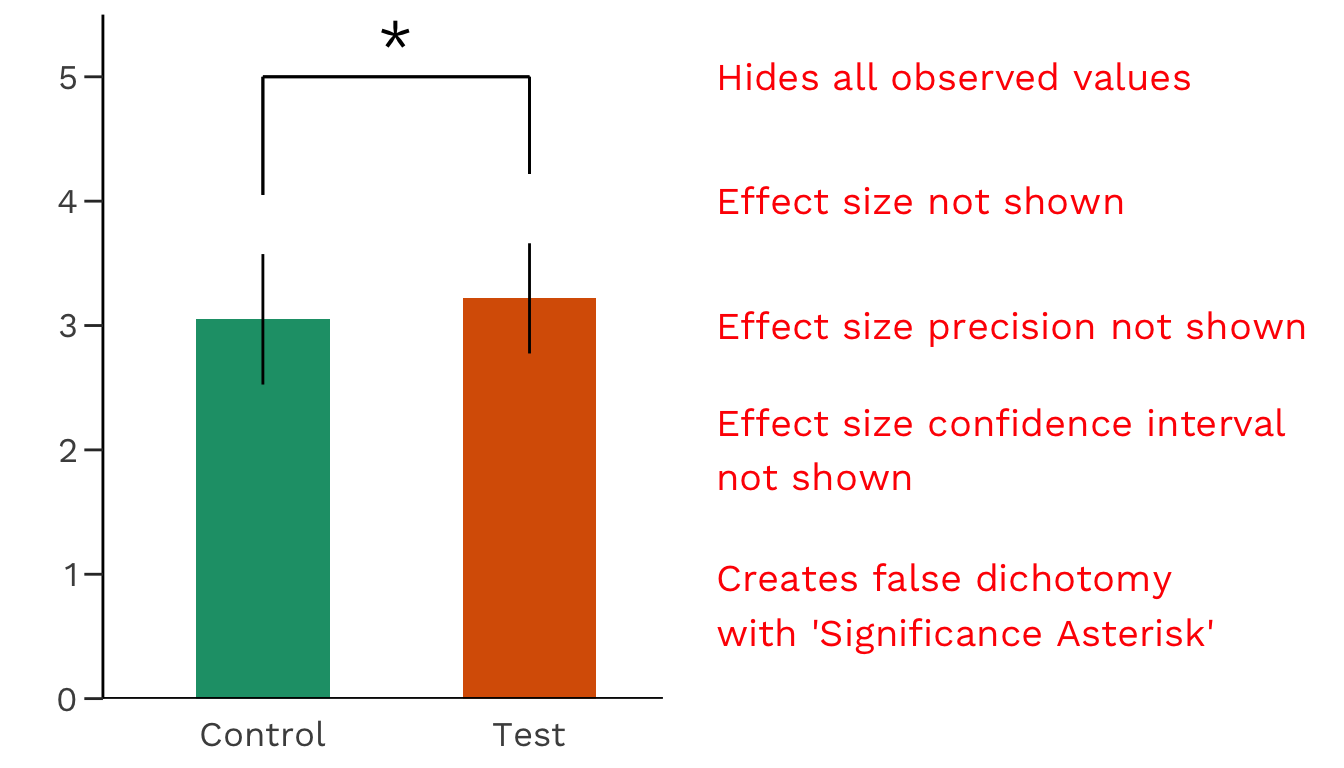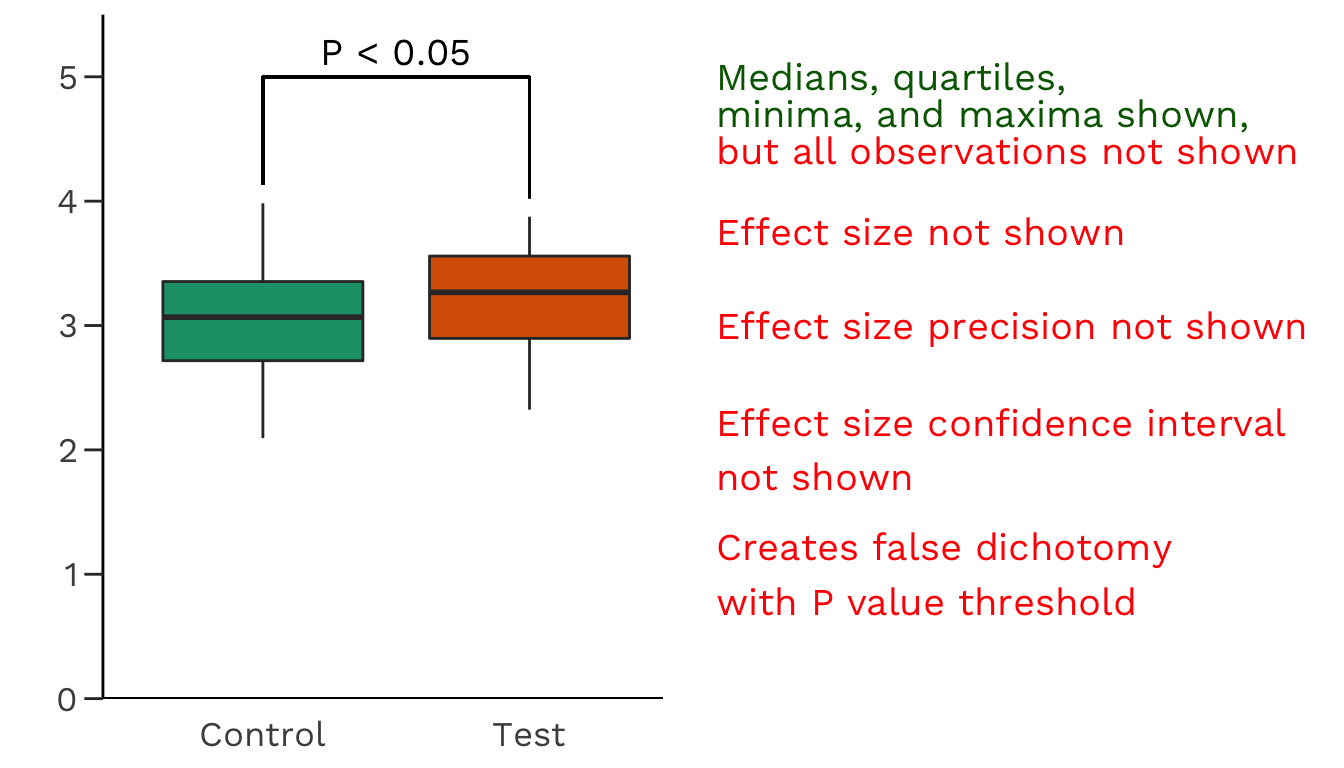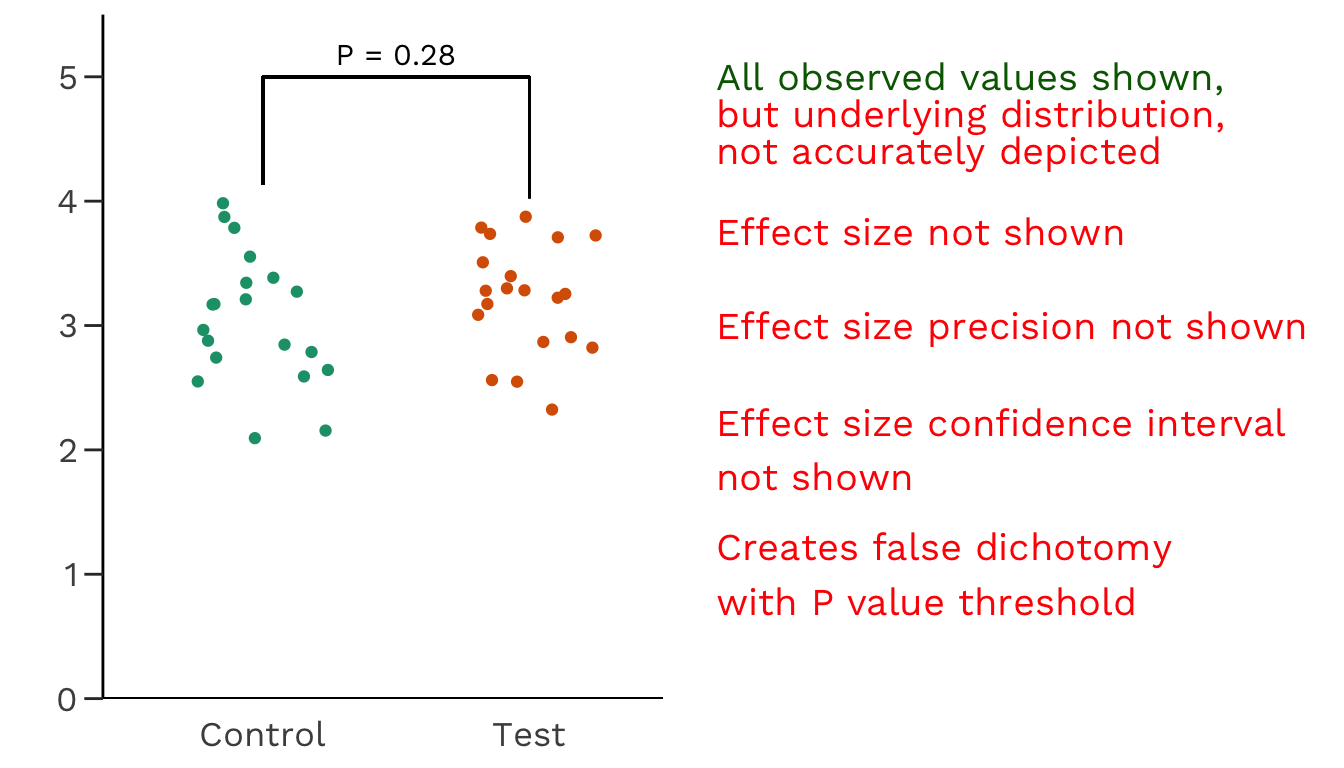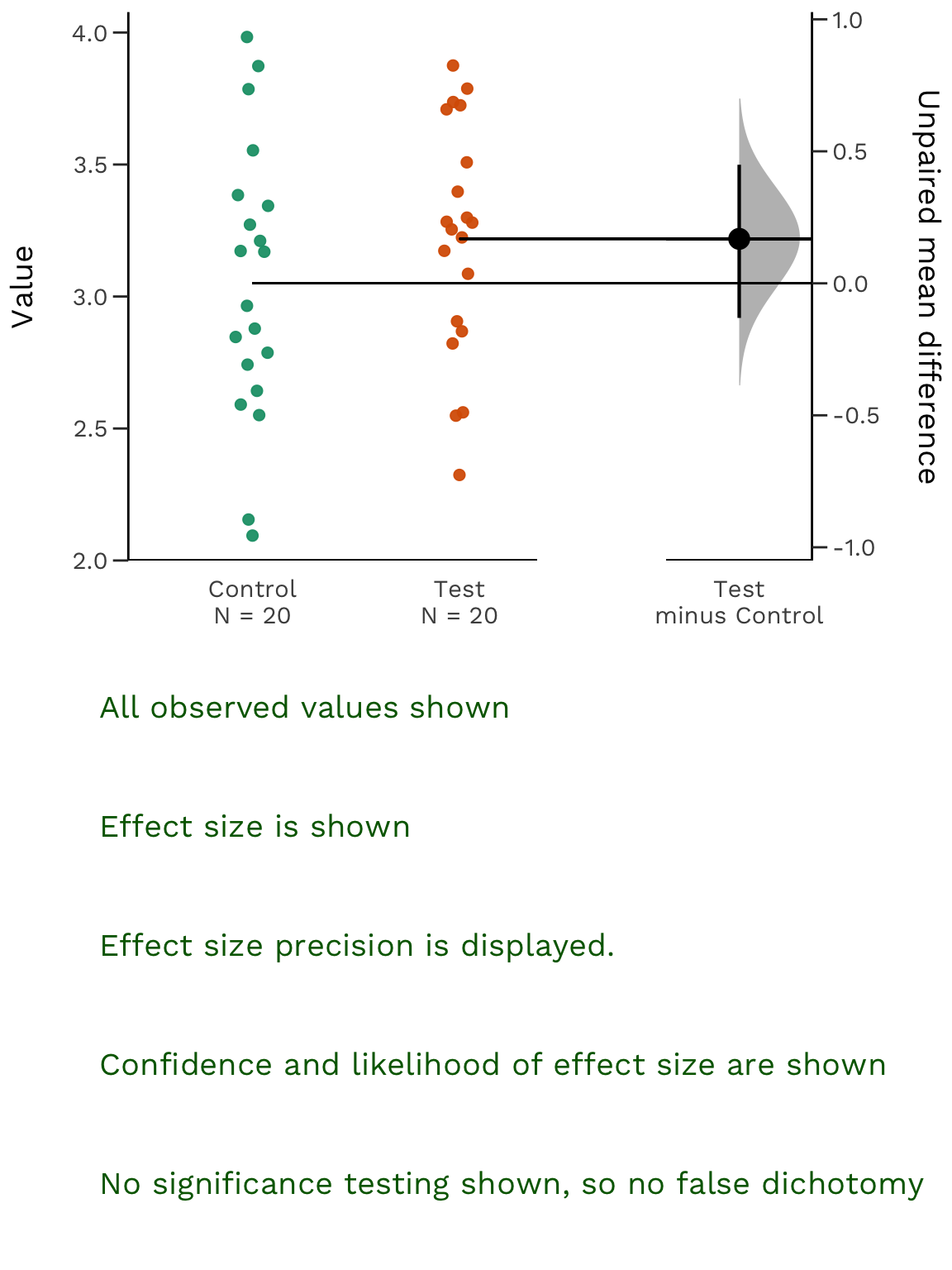# Statistical Visualization

What is data visualization? Battle-Baptiste and Rusert (2018) 1 W. E. B. Du Bois’s Data Portraits: Visualizing Black America. Edited by Whitney Battle-Baptiste and Britt Rusert, Princeton Architectural Press, 2018 give a cogent and compelling definition:

[Data visualization is] the rendering of information in a visual format to help communicate data while also generating new patterns and knowledge through the act of visualization itself.

Sadly, too many figures and visualizations in modern academic publications seemingly fail to “generate new patterns and knowledge through the act of visualization itself”. Here, we propose a solution: the estimation plot.

# The Inadequacy of Common Plots

## The Barplot

Let’s say we have performed an experiment with 20 control subjects, and 20 test subjects. We begin our data analysis by making a barplot of the data.The barplot has several shortcomings, despite enjoying widespread usage in academic journals. We’re not the first ones (see this, this, or that) to point out the myriad flaws with the barplot. Importantly, the barplot does not show us the effect size.

Alternatively, we can use a boxplot to visualize the data.

## The BoxplotUnfortunately, the boxplot still doesn’t show all our data. We still lack information about the underlying distribution of your data. Is it normally distributed? Is there skew in the points? What is the sample size? More importantly, boxplots do not display the effect size.

To display several data points across one or more categories, we can use the jitter plot.

## The Jitter PlotJitter plots avoid overlapping datapoints (i.e. datapoints with the same y-value) by adding a random factor to each point along the orthogonal x-axes. Thus, while a jitter plot displays all datapoints (implicitly indicating the sample size visually), it might not accurately depict the underlying distribution of the data.

# Introducing the Estimation PlotShown above is a Gardner-Altman estimation plot2 The plot draws its name from Martin J. Gardner and Douglas Altman, who are credited with creating the design in 1986.. This plot has two key features. Firstly, it presents all datapoints as a swarmplot, which orders each point to display the underlying distribution. Secondly, an estimation plot presents the effect size as a bootstrap 95% confidence interval (95% CI) on a separate but aligned axes. where the effect size is displayed to the right of the war data, and the mean of the test group is aligned with the effect size. Thus, estimation plots are robust, beautiful, and convey important statistical information elegantly and efficiently.

An estimation plot obtains and displays the 95% CI through nonparametric bootstrap resampling. This enables visualization of the confidence interval as a graded sampling distribution. This is different from the original Gardner-Altman design: the 95% CI was computed through parametric methods, and displayed as a vertical error bar.

## Estimation Statistics

Estimation plots emerge from estimation statistics a simple framework that avoids the pitfalls of significance testing. It focuses on the effect sizes of one’s experiment/interventions, and uses familiar statistical concepts: means, mean differences, and error bars.

Significance testing calculates the probability (the P value) that the experimental data would be observed, if the intervention did not produce a change in the metric measured (i.e. the null hypothesis). This leads analysts to apply a false dichotomy on the experimental intervention.

Estimation statistics, on the other hand, focuses on the magnitude of the effect (the effect size) and its precision. This encourages analysts to gain a deeper understanding of the metrics used, and how they relate to the natural processes being studied.

## An Estimation Plot For Every Experimental Design

For each of the most routine significance tests, there is an estimation replacement:

Unpaired Student’s t-test → Two-group estimation plot